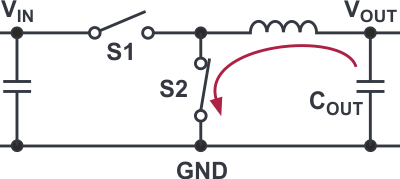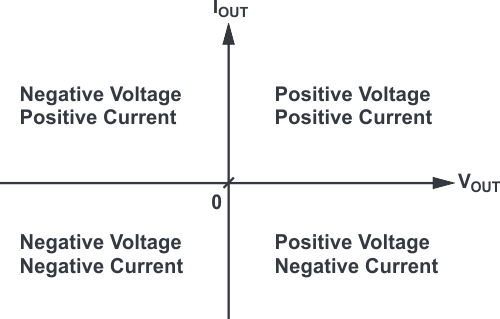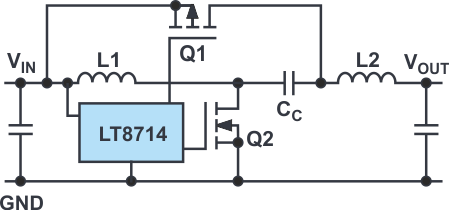# Voltage Conversion in Four Quadrants

## Analog Devices LT8714

A four-quadrant dc-dc converter offers an elegant way to control currents and voltages, and is able to set current to any level.

While simple voltage converters can generate a fixed output voltage from an input voltage, this behavior isn’t sufficient in certain applications. An example is the control of a voltage node that has capacitors connected to it. These capacitors may be charged to any voltage. If they need to be brought to a lower voltage, they have to be partially discharged. Thus, in such an application, a power supply must be able to source or sink current as required.

Such a converter is called a four-quadrant dc-dc converter. For applications of this type, a power supply can be used with an output discharge function. It can quickly discharge output capacitors. Figure 1 shows such a function for a step-down switching regulator. Here, switch S2 is switched on for a lengthy period after the switching action of the buck converter is switched off and the output capacitor is discharged.

An elegant way of controlling currents and voltages is with a four-quadrant dc-dc converter. A typical buck converter only operates in one quadrant. It can generate a positive voltage with a positive current flow; that is, current flowing from the dc-dc converter to the load. With a four-quadrant converter, not only can the output capacitors be discharged, but voltage can also be generated. This is possible because current can flow in the direction of the load or taken away from the load. That’s the case in the previous example about quickly discharging output capacitors.Figure 1. Discharge of an output capacitor via a simple output discharge function.

However, a four-quadrant dc-dc converter can do even more. Besides generating voltages, it can also set the current to any level. This current may be positive or negative. Such four-quadrant converters are frequently used in universal lab power supplies. For example, the user is able to test an LED using a fixed current setting. A solar cell can also be operated, with the four-quadrant converter acting as a load that sinks a predetermined current.

Another interesting application involves tinting window panes with LCD technology. This often requires a precisely set voltage in the positive as well as the negative range to yield a suitable tint level depending on the available light and the desired brightness in the room.

Figure 2 shows what four-quadrant means in relation to a power supply. The quadrants of a coordinate system are shown with voltage on the x-axis and current on the y-axis. Current and voltage can be either positive or negative.Figure 2. Shown is an example of a four-quadrant voltage converter.

The dc-dc converter can thus be used as an energy source and as an energy sink. In other words, the converter can function as a power supply or an electric load.

The LT8714 from Analog Devices, a controller for a four-quadrant regulator, has all of the functions required for this type of regulation. For example, with this IC, it’s possible to precisely maintain a voltage of 0 V. Figure 3 shows a simplified circuit diagram with the controller IC and the power stage. The latter consists of two inductors (L1 and L2), two switches (Q1 and Q2), and the coupling capacitor CC. Explanations on the respective operation in each of the quadrants as well as on the behavior at the transitions from one quadrant to another can be found in the LT8714 datasheet.Figure 3. This simplified circuit diagram illustrates the topology of a four- quadrant voltage converter.

In some applications, a power supply operating in four quadrants is required. With an optimized controller, circuit design is simplified and operation, especially around the frequently critical 0-V crossover point, is clean and reliable.

There are alternatives to using a four-quadrant regulator, such as switching regulator topologies that must be connected in parallel to enable multiple-quadrant functionality. However, these are often more cost-intensive when compared to using a dedicated four-quadrant approach.

## Materials on the topic

electronicdesign.com